# Metric geometry : Pythagorean Theorem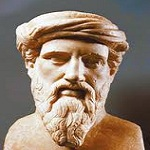The metric geometry is based on the well-known theorem of Pythagoras, to set the metric relationship between the sides of a right triangle.

The concept of the Euclidean space as it adopts in its definition of distance, and geometric relations derived are paramount.

A lesser known Pythagorean theorem we, and recognition to the school of geometers who created, from which we all benefit today.

Pythagoras of Samos (about 582 – 507 to. C., Greek: Pythagoras of Samos) It was a Greek philosopher and mathematician, best known for the Pythagorean Theorem, actually belongs to the Pythagorean school and not only to Pythagoras. His school said "everything is number", thus, was devoted to the study and classification of numbers.(W)

## Statement of the Pythagorean Theorem

In any right triangle the square of the hypotenuse equals the sum of the squares of the legs.(in)

There are several proofs of this important theorem which is the basis of the metric geometry.

The Chou Pei is a mathematical work of dating discussed in some places, although it is accepted that it was written mostly between 500 and 300 to. C.It is believed that Pythagoras did not know this work. Regarding Chui Chang appears to be further, is dated around the year 250 to. C.

The Chou Pei proves the theorem by constructing a square of side (a b) which splits into four triangles base to and altitude b, and a square of side c (W)

Mathematically, it can be stated with the following equation: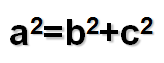This equation states that the area of ​​a square of side “to” is equal to the sum of the areas of two squares, one side “b” and other side “c”. Call itself “to” the hypotenuse (longer side) of a right triangle and “b” and “c” to Hicks, can be represented graphically in the following figure.To show that this equation holds, use two new figures obtained from squares of side “b c”. In the first inscribed a square area whose side to be on this side of the square is drawn. To complete the area of ​​the square of the game we should add four triangles equal rectangles (Light Blue).

In the figure on the right have formed two squares, one side “b” and other side “c”. To complete the total area needed again four right triangles, as in the case previous, which ensures that the square of side “to” has an area equal to the sum of two squares.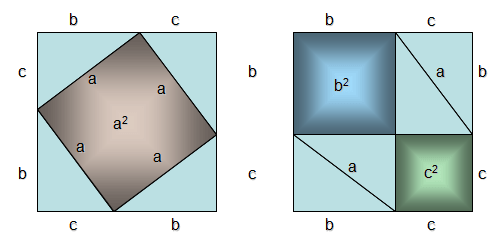This show has the charm of being very graphic and simple, math barely.

## Properties of right triangle

There are two properties of the right triangle (angle is straight) that have special importance for the development of more elaborate such as power and investment that develop models that analyze the concepts tangents are called theorems height and leg.

In the figure is shown a right triangle resting on the hypotenuse. The height of the triangle is the distance from the vertex “A” the hypotenuse (su base).

### Theorems leg and height.

Both theorems are based on the known Thales theorem, establishing a relationship between the sides of two similar triangles.

If two triangles have two equal angles, so is the third. This is because the sum of the internal angles of a triangle always son180 º sexagesimal.

To prove that two triangles are similar enough to show that they have two equal angles.

In the above figure we can find three similar triangles: ABC, ABH y HCA. The three triangles have a right angle, and the share of the angle, then the third is worth the same.

We can therefore, aplicando Thales, establish some equalities as:

BA/BC = BH/BA or AH/HC = BH/AH

BA being the distance between points A and B etc..

The following theorems are obtained directly from the above relations: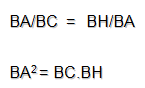Theorem catheter-.The leg of a right triangle is the mean proportional between the hypotenuse and the projection of that leg on the hypotenuse.
• BA is the value of one of the legs,
• Hypotenuse BC
• BH is the projection on the hypotenuse BA
Height theorem-.The height of a right triangle's hypotenuse measure mean proportional between the two segments that divides.
• AH is the measured height of the triangle on the hypotenuse
• BH and the two segments HC dividing the height to the hypotenuse

## Example application of theorem hick

Data (to, b, x. x = a. b ).

Unknown ( Find the mean proportional segment x, between segments of the , b given)## Example of application of the height theorem

Data (to, b, x. x = a. b ).

Unknown ( Find the mean proportional segment x, between segments of the , b given)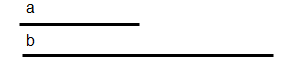Data (m, s, x + y = s , x .y = m. m).

Unknown (Y find two segments known their sum s and its mean proportional mo your product m. m.)## Example of application of right triangle

Given the points A and B. Draw two parallel lines for them to distension magnitude m given.## Self-assessment Test

You must mark V (true) o F (False) each of the following relationships

#### Test 1

For example, a triangle has three heights. If measured from the apex “A” will label with the subscript “to” lowercase.

The opposite sides of a vertex is labeled with the same letter but lower caseTo answer the questions, is recommended to first seek possible relationships derived from applying the theorems presented (cateto y altura).

It is interesting to try to identify graphically each of the items that appear in the equation presented.

Point “H” called foot height hc

H divides the hypotenuse of two segments.

In this case we have misused the designation of the triangle, because you must use the letter “A” for containing the right angle.Remember to graphically identify segments that relate to Figure.

Interest is so graphically form mathematical expressions are not the core training. The graphic constructions are those that should prevail in the learning of basic geometry to achieve high levels of abstraction.